# Unit circle facts for kids

Kids Encyclopedia Facts

In mathematics, a unit circle is a circle with a radius of 1. The equation of the unit circle is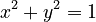$x^2 + y^2 = 1$. The unit circle is centered at the Origin, or coordinates (0,0). It is often used in Trigonometry.

## Trigonometric functions in the unit circle

In a unit circle, where$t$ is the angle desired,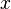$x$ and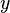$y$ can be defined as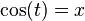$\cos (t) = x$ and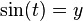$\sin (t) = y$. Using the function of the unit circle,$x^2 + y^2 = 1$, another equation for the unit circle is found,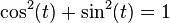$\cos^2(t) + \sin^2(t) = 1$. When working with trigonometric functions, it is mainly useful to use angles with measures between 0 and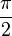$\pi\over 2$ radians, or 0 through 90 degrees. It is possible to have higher angles than that, however. Using the unit circle, two identities can be found: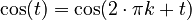$\cos (t) = \cos (2 \cdot \pi k + t)$ and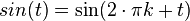$sin (t) = \sin (2 \cdot \pi k + t)$ for any integer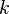$k$.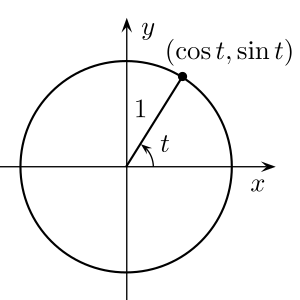The unit circle can substitute variables for trigonometric functions.

## Images for kidsUnit circle Facts for Kids. Kiddle Encyclopedia.